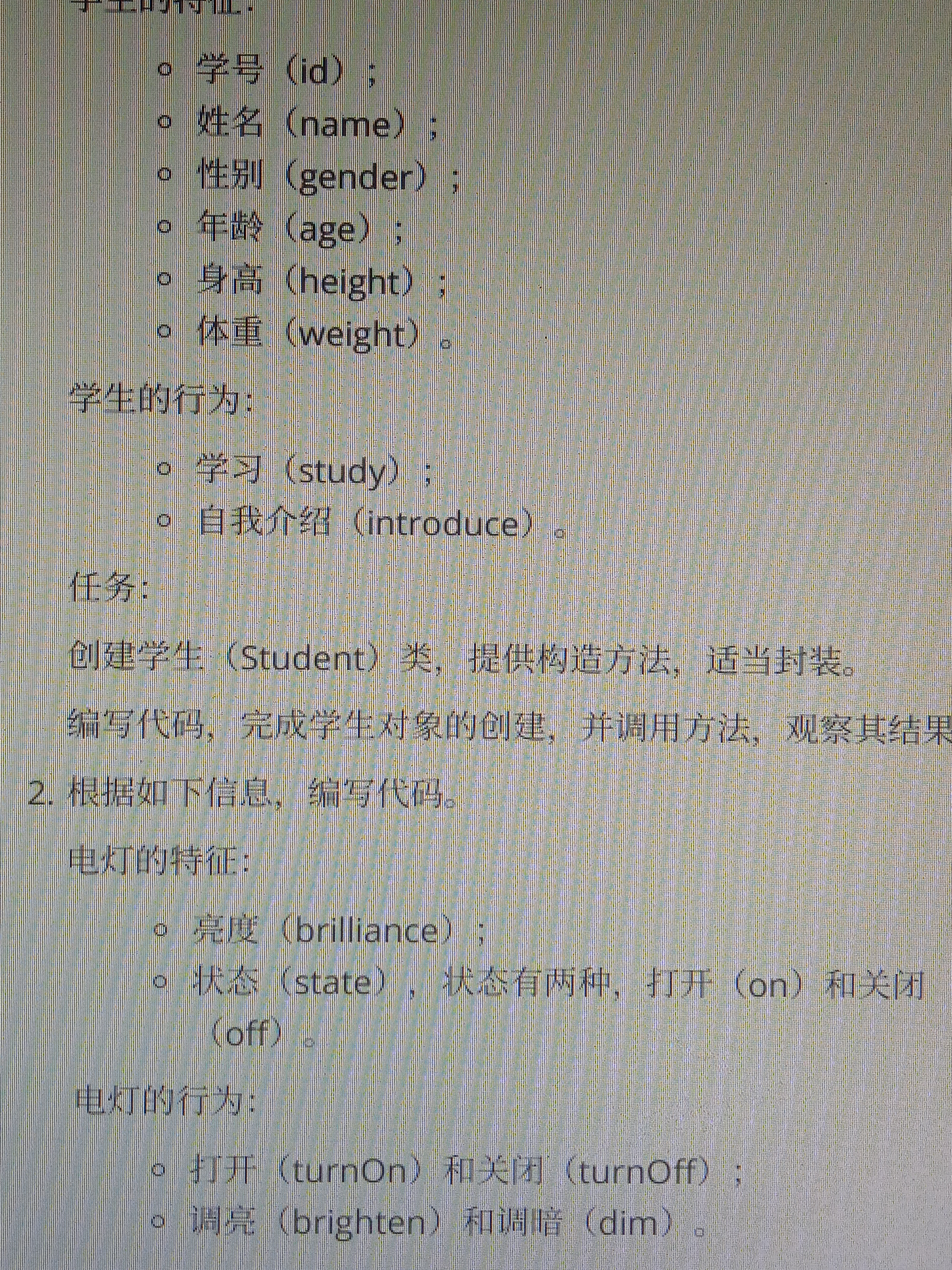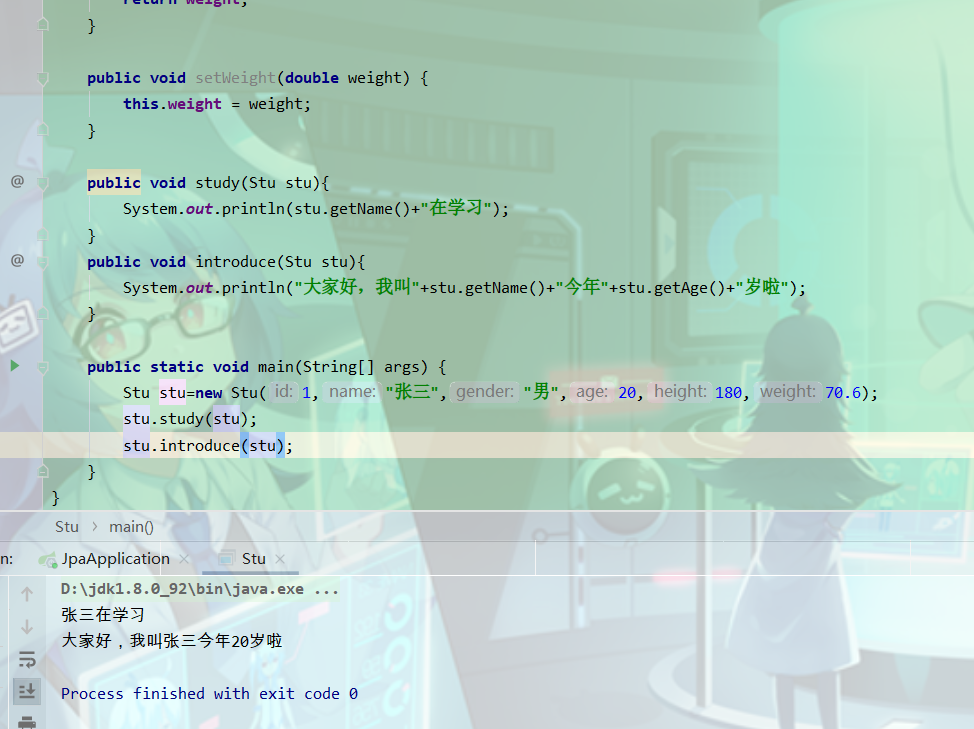2021-06-17 10:44

# 各位大神 解解题 帮帮忙• 点赞
• 写回答
• 关注问题
• 收藏
• 邀请回答

#### 3条回答默认 最新

•CSDN专家-微编程 2021-06-17 11:00
已采纳

第一题代码如下：

``````

public class Stu {
private int id;
private String name;
private String gender;
private int age;
private double height;
private double weight;

public Stu() {
}

public Stu(int id, String name, String gender, int age, double height, double weight) {
this.id = id;
this.name = name;
this.gender = gender;
this.age = age;
this.height = height;
this.weight = weight;
}

public int getId() {
return id;
}

public void setId(int id) {
this.id = id;
}

public String getName() {
return name;
}

public void setName(String name) {
this.name = name;
}

public String getGender() {
return gender;
}

public void setGender(String gender) {
this.gender = gender;
}

public int getAge() {
return age;
}

public void setAge(int age) {
this.age = age;
}

public double getHeight() {
return height;
}

public void setHeight(double height) {
this.height = height;
}

public double getWeight() {
return weight;
}

public void setWeight(double weight) {
this.weight = weight;
}

public void study(Stu stu){
System.out.println(stu.getName()+"在学习");
}
public void introduce(Stu stu){
System.out.println("大家好，我叫"+stu.getName()+"今年"+stu.getAge()+"岁啦");
}

public static void main(String[] args) {
Stu stu=new Stu(1,"张三","男",20,180,70.6);
stu.study(stu);
stu.introduce(stu);
}
}
``````

运行截图：第二题和第一题一样套路，如果对你有帮助记得采纳一下，谢谢

点赞 1 评论
•CSDN专家-sinjack 2021-06-17 10:45

我帮你写，需要点时间。

点赞 评论
•CSDN专家-微编程 2021-06-17 10:50

你这个应该是面向对象的那一节吧，创建对象，设置属性，然后封装，生成构造方法，编写自定义方法，编写主函数main，调用构造方法创建对象，然后调用对象.自定义方法()  即可

点赞 评论